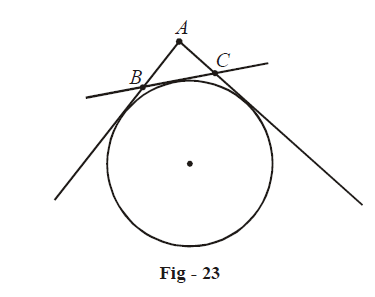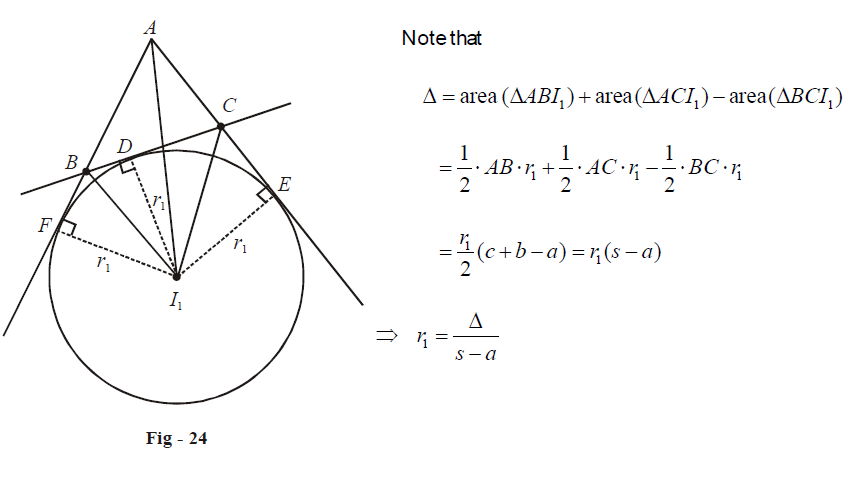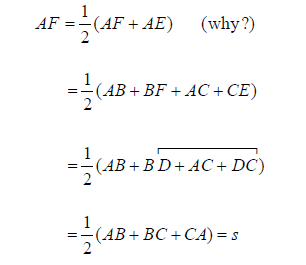# Excircle Formulae

Let us now turn our attention to excircles. For any triangle $$\Delta ABC$$ , there will be three ex-circles. For examples, the ex-circle opposite to the angle A will touch the side BC and two sides AB and AC produced:The ex-circle opposite to the angle A. The radius of this circle will be denoted by r1. Similarly, the radii of the other two circles are denoted by r2 and r3.

The next four relations are concerned with the ex-radii  $${r_1},{r_2},{r_3}$$  and how these relate to the other parameters of the triangle:

\boxed{\begin{align} & \ {{r}_{1}}=\frac{\Delta }{s-a}, & &{{r}_{2}}=\frac{\Delta }{s-b},& &{{r}_{3}}=\frac{\Delta }{s-c}\ \\ & \ {{r}_{1}}=s\tan \frac{A}{2}, & &{{r}_{2}}=s\tan \frac{B}{2},& &{{r}_{3}}=s\tan \frac{C}{2}\ \\ & \ {{r}_{1}}=\frac{a\cos \frac{B}{2}\cos \frac{C}{2}}{\cos \frac{A}{2}},& &{{r}_{2}}=\frac{b\cos \frac{C}{2}\cos \frac{A}{2}}{\cos \frac{B}{2}},& &{{r}_{3}}=\frac{c\cos \frac{A}{2}\cos \frac{B}{2}}{\cos \frac{C}{2}}\ \\ & \ {{r}_{1}}=4R\sin \frac{A}{2}\cos \frac{B}{2}\cos \frac{C}{2},& &{{r}_{2}}=4R\cos \frac{A}{2}\sin \frac{B}{2}\cos \frac{C}{2},& &{{r}_{3}}=4R\cos \frac{A}{2}\cos \frac{B}{2}\sin \frac{C}{2} \end{align}}

Let us justify the first of each set of relations.Similarly, \begin{align}{r_2} = \frac{\Delta }{{s - b}}\;{\text{and}}\;{r_3} = \frac{\Delta }{{s - c}}\end{align}

Now, let us consider the second set of relations. In  $$\Delta AFI$$  , we’ll have  $${r_1} = AF\tan \frac{A}{2}$$ . We need to prove that AF = s. To that end;Thus,  \begin{align}{r_1} = s\tan \frac{A}{2}\end{align}  . Similarly, we can show that  \begin{align}{{r}_{2}}=s\tan \frac{B}{2}\;\;\;and\;\;\;{{r}_{3}}=s\tan \frac{C}{2}\text{ }\!\!~\!\!\text{ }.\end{align}

To prove the third set of relations, we note from  $$\Delta {I_1}BD$$  and  $$\Delta {I_1}CD$$  that

\begin{align}&\;\;\,\,\,\,\,\,\,\,\,\,\,\,\,\,\,\,\,\,BD = {r_1}\tan \frac{B}{2},\;\;\;\;\;DC = {r_1}\tan \frac{C}{2} & \left\{ {{\text{Justify}}\;{\text{these relations}}\;{\text{carefully}}} \right\} \\ &\Rightarrow\qquad BD + DC = BC = a = {r_1}\left( {\tan \frac{B}{2} + \tan \frac{C}{2}} \right) \\ &\Rightarrow\qquad {r_1} = \frac{a}{{\tan \frac{B}{2} + \tan \frac{C}{2}}} \\ \,\,\,\,\,\,\,\,\,\,\,\,\,\,\,\,\,\,\, &\qquad\qquad\;= \frac{{a\cos \frac{B}{2}\cos \frac{C}{2}}}{{\sin \left( {\frac{{B + C}}{2}} \right)}} \\ \,\,\,\,\,\,\,\,\,\,\,\,\,\,\,\, &\qquad\qquad\;= \frac{{a\cos \frac{B}{2}\cos \frac{C}{2}}}{{\cos \frac{A}{2}}} \\ \end{align}

Similar relations hold for $${r_2}\;{\text{and}}\;{r_3}$$  .

Finally, to prove the fourth set of relations, we use the relations  $$a = 2R\sin A$$  etc, so that

\begin{align}& {{r}_{1}}=\frac{2R\sin A\cos \frac{B}{2}\cos \frac{C}{2}}{\cos \frac{A}{2}} \\ &\quad =4R\sin \frac{A}{2}\cos \frac{B}{2}\cos \frac{C}{2} \end{align}

and similarly, for  $${r_2}\;{\text{and}}\;{r_3}$$ .